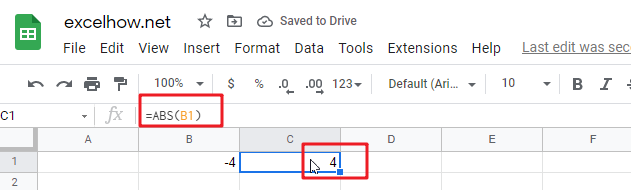# ExcelHow

This post will guide you how to use Google Sheets ABS function with syntax and examples.

## Description

The Google Sheets ABS function returns the absolute value of a number.

The ABS function can be used to convert negative numbers to positive numbers in google sheets. The purpose of this function is to get the absolute value of a given number and its returned value is positive number.

The ABS function is a build-in function in Google Sheets and it is categorized as a MATH function.

## Syntax

The syntax of the ABS function is as below:

=ABS (number)

Where the ABS function argument is:

• Number – This is a required argument.  A number that you want the absolute value

Note: ABS function only takes one argument, it must be a numeric value. If argument is not a numeric value, ABS function returns a #VALUE! Error.

## Google Sheets ABS Function Examples

The below examples will show you how to use google sheets ABS function to return the absolute value of a number.

#1 =ABS(B1)